# SAS/STAT Examples

## Bayesian Linear Regression with Standardized Covariates

Contents | SAS Program | PDF

## Overview

This example uses the MCMC procedure to fit a Bayesian linear regression model with standardized covariates. It shows how the random walk Metropolis sampling algorithm struggles when the scales of the regression parameters are vastly different. It also illustrates that the sampling algorithm performs quite well when the covariates are standardized.

## Analysis

The following data set contains salary and performance information for Major League Baseball players (excluding pitchers) who played at least one game in both the 1986 and 1987 seasons. The salaries are for the 1987 season (Time Inc.; 1987 ) , and the performance measures are from the 1986 season (Reichler; 1987 ) .

```data baseball;
input logSalary no_hits no_runs no_rbi no_bb yr_major cr_hits @@;
yr_major2 = yr_major*yr_major;
cr_hits2  = cr_hits*cr_hits;
label no_hits="Hits in 1986" no_runs="Runs in 1986"
no_rbi="RBIs in 1986"  no_bb="Walks in 1986"
yr_major="Years in MLB" cr_hits="Career Hits"
yr_major2="Years in MLB^2" cr_hits2="Career Hits^2"
logSalary = "log10(Salary)";
datalines;
.  66 30 29 14  1   66
2.6766936096  81 24 38 39 14  835

... more lines ...

2.84509804 127 65 48 37  5  806
2.942008053 136 76 50 94 12 1511
2.5854607295 126 61 43 52  6  433
2.982271233 144 85 60 78  8  857
3 170 77 44 31 11 1457
;
```

The MEANS procedure produces summary statistics for these data. Summary measures are saved to the SUM_BASEBALL data set for future analysis.

```proc means data = baseball mean stddev;
output out=sum_baseball(drop=_type_ _freq_);
run;
```

Figure 1 displays the results.

Figure 1 PROC MEANS Summary
The MEANS Procedure

Variable Label Mean Std Dev
logSalary
no_hits
no_runs
no_rbi
no_bb
yr_major
cr_hits
yr_major2
cr_hits2
log10(Salary)
Hits in 1986
Runs in 1986
RBIs in 1986
Walks in 1986
Years in MLB
Career Hits
Years in MLB^2
Career Hits^2
 2.57301 103.558 52.3333 49.4486 39.8879 7.62928 736.757 82.0717 933095
 0.38646 44.1548 25.0098 25.5045 21.1217 4.89288 625.702 93.8925 1.37113e+06

## Bayesian Linear Regression Model

Suppose you want to fit a Bayesian linear regression model for the logarithm of a player’s salary with density as follows: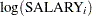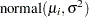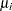( 1 )

where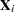is the vector of covariates listed as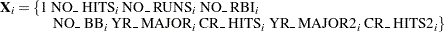for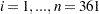baseball players. Pete Rose was an extreme outlier in 1986, and his information greatly skews results. He is omitted from this data set and analysis.

The likelihood function for the logarithm of salary and the corresponding covariates is( 2 )

wheredenotes a conditional probability density. The normal density is evaluated at the specified value ofand the corresponding mean parameter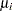defined in Equation 1 . The regression parameters in the likelihood are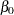through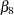.

Suppose the following prior distributions are placed on the parameters: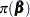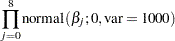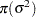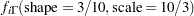( 3 )

where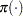indicates a prior distribution andis the density function for the inverse-gamma distribution. Priors of this type with large variances are often called diffuse priors.

Using Bayes’ theorem, the likelihood function and prior distributions determine the posterior distribution of the parameters as follows: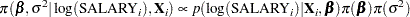PROC MCMC obtains samples from the desired posterior distribution. You do not need to specify the exact form of the posterior distribution.

The following SAS statements use the likelihood function and prior distributions to fit the Bayesian linear regression model. The PROC MCMC statement invokes the procedure and specifies the input data set. The NBI= option specifies the number of burn-in iterations. The NMC= option specifies the number of posterior simulation iterations. The SEED= option specifies a seed for the random number generator (the seed guarantees the reproducibility of the random stream). The PROPCOV=QUANEW option uses the estimated inverse Hessian matrix as the initial proposal covariance matrix.

```ods graphics on;
proc mcmc data=baseball nbi=50000 nmc=10000 seed=1181 propcov=quanew;
array beta beta0-beta8;
array data 1 no_hits  no_runs  no_rbi no_bb
yr_major cr_hits yr_major2 cr_hits2;

parms beta: 0;
parms sig2 1;

prior beta: ~ normal(0,var = 1000);
prior sig2 ~ igamma(shape = 3/10, scale = 10/3);

call mult(beta, data, mu);
model logsalary ~ n(mu, var = sig2);
run;
ods graphics off;
```

Each of the two ARRAY statements associates a name with a list of variables and constants. The first ARRAY statement specifies names for the regression coefficients. The second ARRAY statement contains all of the covariates.

The first PARMS statement places all regression parameters in a single block and assigns them an initial value of 0. The second PARMS statement places the variance parameter in a separate block and assigns it an initial value of 1.

The first PRIOR statement assigns the normal prior to each of the regression parameters. The second PRIOR statement assigns the inverse-gamma prior distribution to.

The CALL statement uses the MULT matrix multiplication function to calculate. The MODEL statement specifies the likelihood function as given in Equation 2 .

The first step in evaluating the results is to review the convergence diagnostics. With ODS Graphics turned on, PROC MCMC produces graphs. Figure 2 displays convergence diagnostic graphs for theregression parameter. The trace plot indicates that the chain does not appear to have reached a stationary distribution and appears to have poor mixing. The diagnostic plots for the rest of the parameters (not shown here) tell a similar story.

Figure 2 Bayesian Diagnostic Plots forThe non-convergence exhibited here results because the parameters are scaled very differently from each other for these data. The random walk Metropolis algorithm is not an optimal sampling algorithm in the case where the parameters have vastly different scales. Standardized covariates (Mayer and Younger; 1976 ) eliminate this problem, and the random walk Metropolis algorithm proceeds smoothly.

## Bayesian Linear Regression Model with Standardized Covariates

Suppose you want to fit the same Bayesian linear regression model, but you want to use standardized covariates. You rewrite the mean function in Equation 1 as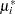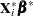where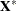is the design matrix constructed from a column of 1s andstandardized covariates. The regression parameters on the standardized scale are represented by. The standardized covariates are computed as follows: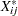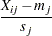forplayers andcovariates, and where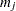andare the mean and standard deviation of theth covariate, respectively.

The following statements manipulate the SUM_BASEBALL output data set from the earlier use of PROC MEANS. The statements create macro variables for the means and standard deviations to use later in the analysis. The macro variables are independent of SAS data set variables and can be referenced in SAS procedures to facilitate computations. The TRANSPOSE procedure transposes the SUM_BASEBALL data set and a DATA step creates the macro variables by using the SYMPUTX functions. The %PUT statements enable you to verify that the macro variables have been created successfully.

```proc transpose data=sum_baseball out=tab;
id _stat_;
run;

data _null_;
set tab;
sub = put((_n_-1), 1.);
call symputx(compress('m' || sub,'*'), mean);
call symputx(compress('s' || sub,'*'), std);
run;

%put &m1 &m2 &m3 &m4 &m5 &m6 &m7 &m8;
%put &s1 &s2 &s3 &s4 &s5 &s6 &s7 &s8;
```

In this example,andwere calculated in the MEANS procedure and recorded in the macro variables M1–M8 and S1–S8, respectively. The STANDARD procedure computes standardized values of the variables in the original data set.

```proc standard data=baseball out=baseball_std mean=0 std=1;
var no_hits -- cr_hits2;
run;
```

The new likelihood function for the logarithm of the salary and corresponding standardized covariates is as follows: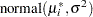For ease of interpretation and inference, you can transform the standardized regression parameters back to the original scale with the following formulas: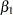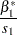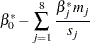Suppose the following diffuse prior distribution is placed on: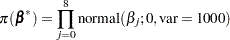The prior distribution foris given in Equation 3 .

Using Bayes' theorem, the likelihood function and prior distributions determine the posterior distribution of the parameters as follows: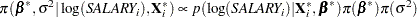The following SAS statements fit the Bayesian linear regression model. The MONITOR= option outputs analysis on selected symbols of interest in the program.

```ods graphics on;
proc mcmc data=baseball_std nbi=10000 nmc=20000 seed=1181
propcov=quanew monitor=(beta0-beta8 sig2);
array beta beta0-beta8 (0);
array betastar betastar0-betastar8;
array data 1 no_hits  no_runs  no_rbi no_bb
yr_major cr_hits yr_major2 cr_hits2;
array mn (0 &m1 &m2 &m3 &m4 &m5 &m6 &m7 &m8);
array std (0 &s1 &s2 &s3 &s4 &s5 &s6 &s7 &s8);

parms betastar: 0;
parms sig2 1;

prior betastar: ~ normal(0,var = 1000);
prior sig2 ~ igamma(shape = 3/10, scale = 10/3);

call mult(betastar, data, mu);
model logsalary ~ n(mu, var = sig2);

beginprior;
summ = 0;
do i = 2 to 9;
beta[i] = betastar[i]/std[i];
summ = summ + beta[i]*mn[i];
end;
beta0 = betastar0 - summ;
endprior;
run;
ods graphics off;
```

The first two ARRAY statements specify names for the regression coefficients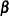andfor the original and standardized scale, respectively. The last three ARRAY statements for DATA, MN, and STD vectors take advantage of PROC MCMC’s ability to use both matrix functions and the SAS programming language. The PARMS, PRIOR, and MODEL statements are called with the same syntax as in the first call to the MCMC procedure.

The BEGINPRIOR and ENDPRIOR statements reduce unnecessary observation-level computations. The statements inside the BEGINPRIOR and ENDPRIOR statements create a block of statements that are run only once per iteration rather than once for each observation at each iteration. This enables a quick update of the symbols enclosed in the statements. The statements within the BEGINPRIOR and ENDPRIOR block transform thesampled values back to.

The trace plot in Figure 3 indicates that the chain appears to have reached a stationary distribution. It also has good mixing and is dense. The autocorrelation plot indicates low autocorrelation and efficient sampling. Finally, the kernel density plot shows the smooth, unimodal shape of posterior marginal distribution for. The remaining diagnostic plots (not shown here) similarly indicate good convergence in the other parameters. Using standardized covariates solves the case of convergence for this model for these data.

Figure 3 Bayesian Diagnostic Plots forUsing Standardization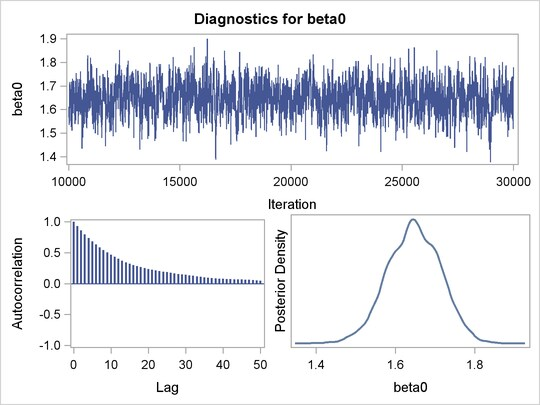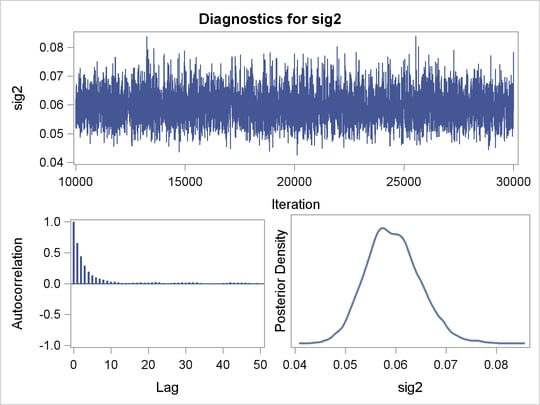Figure 4 reports summary and interval statistics of all parameters. For example, the mean salary increases by an estimated factor of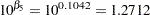(approximately 27%) for each year the player was in Major League Baseball. Similarly, using the same formula,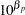, you can see how the mean salary changes by one unit for each of thecovariates. Both the equal tail and highest posterior density (HPD) intervals include 0 for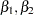, and, indicating that the change in salary with respect to these covariates is not significant. The number of years played seems to be the most influential covariate, followed by the number of career hits.

Figure 4 Posterior Model Summary of Bayesian Linear Regression with Standardized Covariates

The MCMC Procedure

Posterior Summaries
Parameter N Mean Standard
Deviation
Percentiles
25% 50% 75%
beta0 20000 1.6465 0.0666 1.6006 1.6470 1.6935
beta1 20000 -0.00007 0.000938 -0.00071 -0.00004 0.000594
beta2 20000 0.000882 0.00167 -0.00023 0.000860 0.00200
beta3 20000 0.00186 0.000993 0.00119 0.00186 0.00253
beta4 20000 0.00218 0.000980 0.00152 0.00217 0.00281
beta5 20000 0.1042 0.0205 0.0902 0.1038 0.1176
beta6 20000 0.000748 0.000163 0.000642 0.000750 0.000857
beta7 20000 -0.00629 0.000978 -0.00692 -0.00629 -0.00562
beta8 20000 -1.46E-7 5.867E-8 -1.86E-7 -1.47E-7 -1.08E-7
sig2 20000 0.0595 0.00533 0.0558 0.0592 0.0629

Posterior Intervals
Parameter Alpha Equal-Tail Interval HPD Interval
beta0 0.050 1.5123 1.7714 1.5120 1.7705
beta1 0.050 -0.00195 0.00165 -0.00192 0.00168
beta2 0.050 -0.00235 0.00417 -0.00233 0.00418
beta3 0.050 -0.00006 0.00382 -0.00001 0.00383
beta4 0.050 0.000236 0.00412 0.000303 0.00416
beta5 0.050 0.0651 0.1450 0.0625 0.1415
beta6 0.050 0.000428 0.00107 0.000428 0.00107
beta7 0.050 -0.00827 -0.00443 -0.00822 -0.00442
beta8 0.050 -2.62E-7 -3.17E-8 -2.63E-7 -3.32E-8
sig2 0.050 0.0498 0.0705 0.0494 0.0699

## References

Mayer, L. S. and Younger, M. S. (1976), “Estimation of Standardized Regression Coefficients,” Journal of the American Statistical Association , 71(353), 154–157.

Reichler, J. L., ed. (1987), The 1987 Baseball Encyclopedia Update , New York: Macmillan.

Time Inc. (1987), “What They Make,” Sports Illustrated , 54–81.